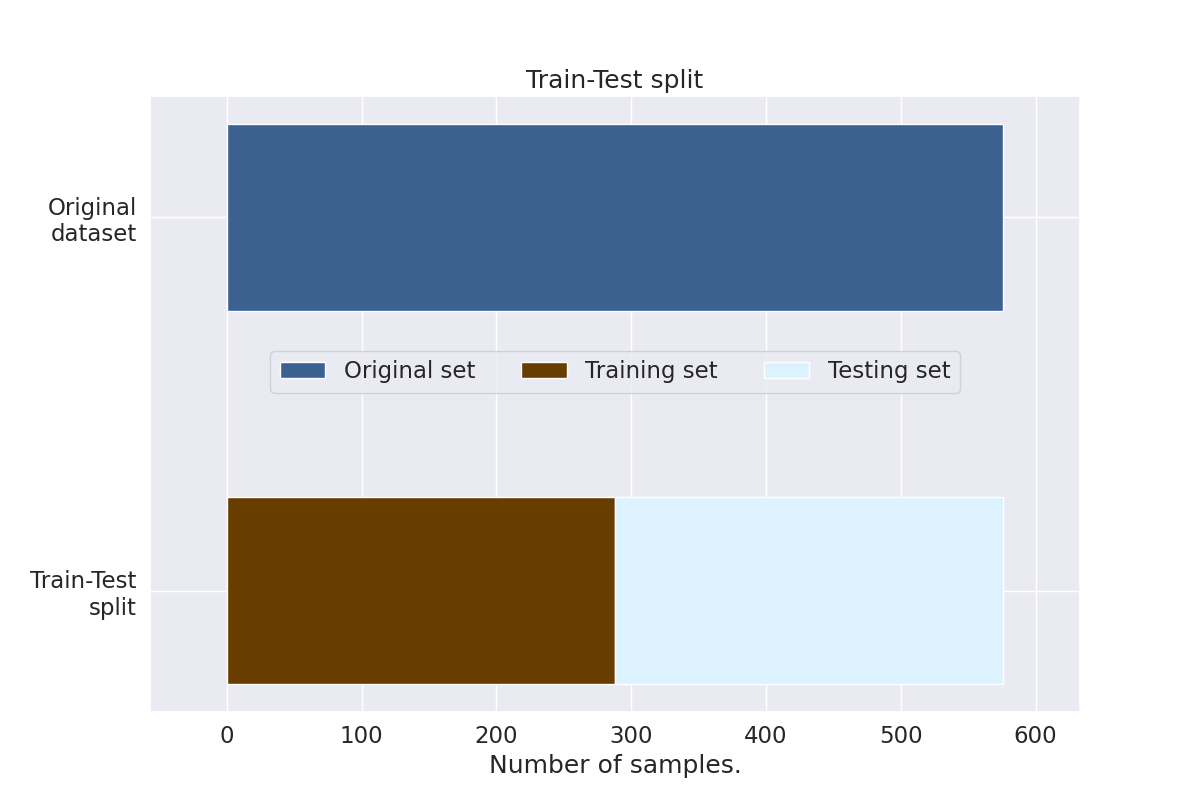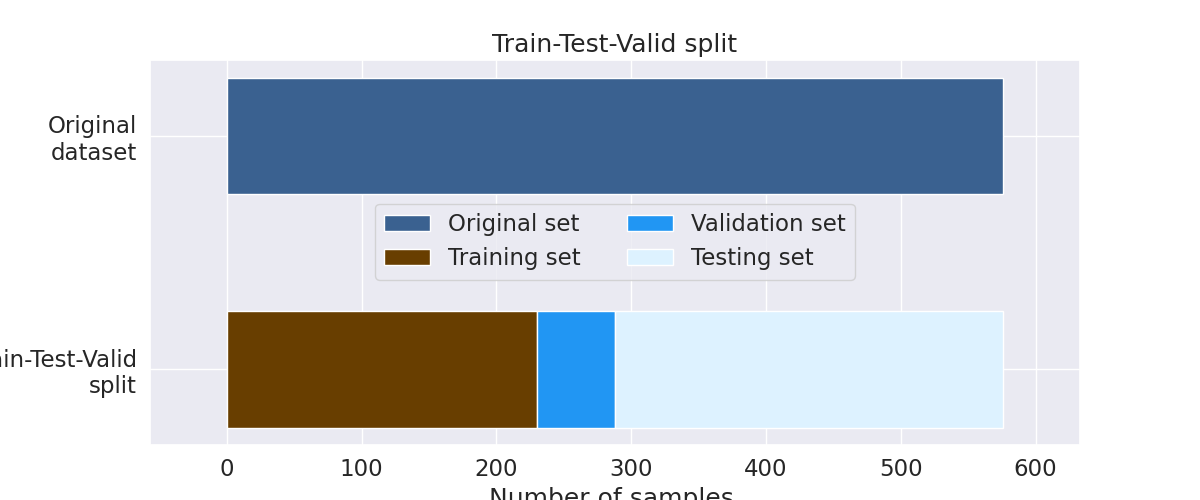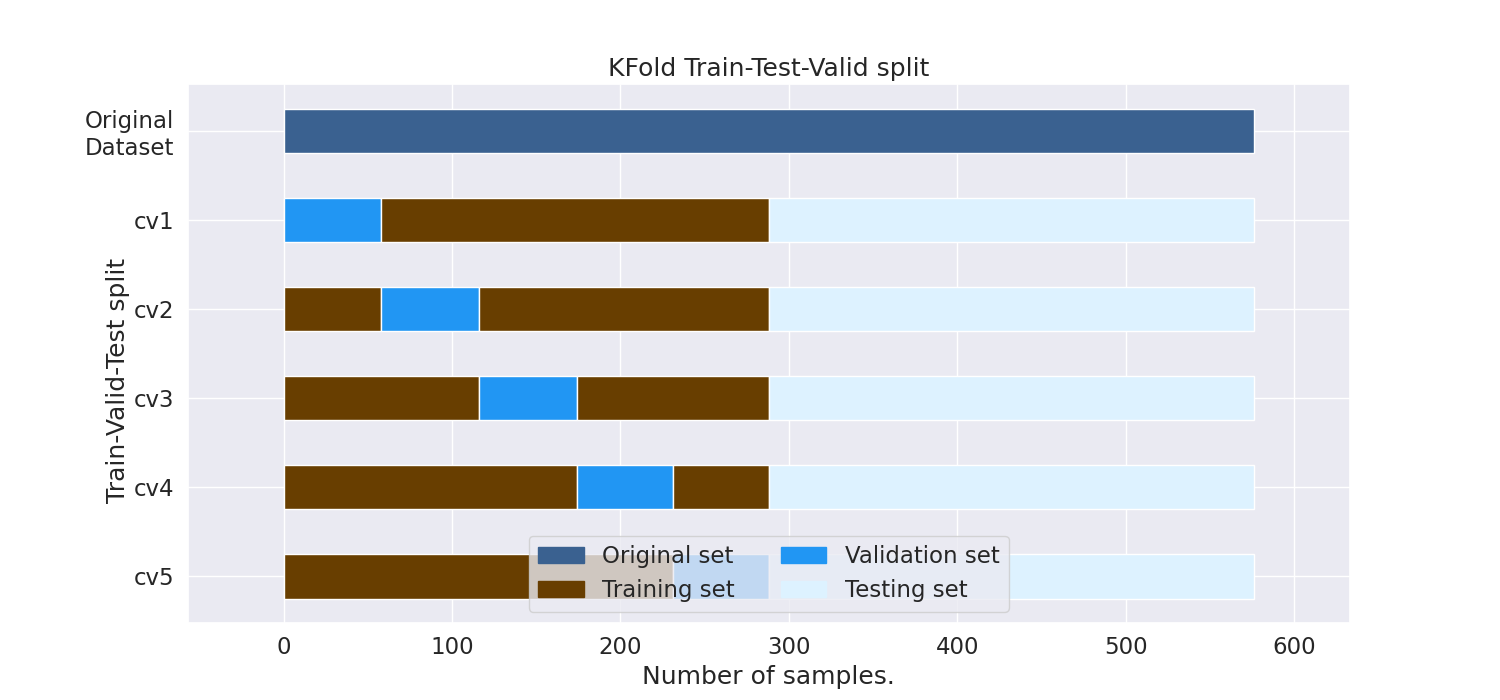# How to train, test and tune your model#

This tutorial shows you how to properly train, tune and test your deep learning models with Braindecode. We will use the BCIC IV 2a dataset as a showcase example.

The methods shown can be applied to any standard supervised trial-based decoding setting. This tutorial will include additional parts of code like loading and preprocessing, defining a model, and other details which are not exclusive to this page (compare Cropped Decoding Tutorial). Therefore we will not further elaborate on these parts and you can feel free to skip them.

In general we distinguish between “usual” training and evaluation and hyperparameter search. The tutorial is therefore split into two parts, one for the three different training schemes and one for the two different hyperparameter tuning methods.

## Why should I care about model evaluation?#

Short answer: To produce reliable results!

In machine learning, we usually follow the scheme of splitting the data into two parts, training and testing sets. It sounds like a simple division, right? But the story does not end here.

While developing a ML model you usually have to adjust and tune hyperparameters of your model or pipeline (e.g., number of layers, learning rate, number of epochs). Deep learning models usually have many free parameters; they could be considered complex models with many degrees of freedom. If you kept using the test dataset to evaluate your adjustmentyou would run into data leakage.

This means that if you use the test set to adjust the hyperparameters of your model, the model implicitly learns or memorizes the test set. Therefore, the trained model is no longer independent of the test set (even though it was never used for training explicitly!). If you perform any hyperparameter tuning, you need a third split, the so-called validation set.

This tutorial shows the three basic schemes for training and evaluating the model as well as two methods to tune your hyperparameters.

Warning

You might recognize that the accuracy gets better throughout the experiments of this tutorial. The reason behind that is that we always use the same model with the same paramters in every segment to keep the tutorial short and readable. If you do your own experiments you always have to reinitialize the model before training.

```from braindecode.datasets import MOABBDataset

subject_id = 3
dataset = MOABBDataset(dataset_name="BNCI2014001", subject_ids=[subject_id])
```

### Preprocessing#

```import numpy as np

from braindecode.preprocessing import (
exponential_moving_standardize,
preprocess,
Preprocessor,
)

low_cut_hz = 4.0  # low cut frequency for filtering
high_cut_hz = 38.0  # high cut frequency for filtering
# Parameters for exponential moving standardization
factor_new = 1e-3
init_block_size = 1000

preprocessors = [
Preprocessor("pick_types", eeg=True, meg=False, stim=False),  # Keep EEG sensors
Preprocessor(
lambda data, factor: np.multiply(data, factor),  # Convert from V to uV
factor=1e6,
),
Preprocessor("filter", l_freq=low_cut_hz, h_freq=high_cut_hz),  # Bandpass filter
Preprocessor(
exponential_moving_standardize,  # Exponential moving standardization
factor_new=factor_new,
init_block_size=init_block_size,
),
]

# Transform the data
preprocess(dataset, preprocessors)
```
```/home/runner/work/braindecode/braindecode/braindecode/preprocessing/preprocess.py:55: UserWarning: Preprocessing choices with lambda functions cannot be saved.
warn('Preprocessing choices with lambda functions cannot be saved.')

<braindecode.datasets.moabb.MOABBDataset object at 0x7f50b213ba90>
```

### Cut Compute Windows#

```from braindecode.preprocessing import create_windows_from_events

trial_start_offset_seconds = -0.5
# Extract sampling frequency, check that they are same in all datasets
sfreq = dataset.datasets.raw.info["sfreq"]
assert all([ds.raw.info["sfreq"] == sfreq for ds in dataset.datasets])
# Calculate the trial start offset in samples.
trial_start_offset_samples = int(trial_start_offset_seconds * sfreq)

# Create windows using braindecode function for this. It needs parameters to define how
# trials should be used.
windows_dataset = create_windows_from_events(
dataset,
trial_start_offset_samples=trial_start_offset_samples,
trial_stop_offset_samples=0,
)
```

### Create model#

```import torch
from braindecode.util import set_random_seeds
from braindecode.models import ShallowFBCSPNet

cuda = torch.cuda.is_available()  # check if GPU is available, if True chooses to use it
device = "cuda" if cuda else "cpu"
if cuda:
torch.backends.cudnn.benchmark = True
seed = 20200220
set_random_seeds(seed=seed, cuda=cuda)

n_classes = 4
# Extract number of chans and time steps from dataset
n_channels = windows_dataset.shape
input_window_samples = windows_dataset.shape

model = ShallowFBCSPNet(
n_channels,
n_classes,
input_window_samples=input_window_samples,
final_conv_length="auto",
)

# Send model to GPU
if cuda:
model.cuda()
```

## How to train and evaluate your model#

### Split dataset into train and test#

We can easily split the dataset using additional info stored in the description attribute, in this case the `session` column. We select `session_T` for training and `session_E` for testing. For other datasets, you might have to choose another column.

Note

No matter which of the three schemes you use, this initial two-fold split into train_set and test_set always remains the same. Remember that you are not allowed to use the test_set during any stage of training or tuning.

```splitted = windows_dataset.split("session")
train_set = splitted["session_T"]
test_set = splitted["session_E"]
```

### Option 1: Simple Train-Test Split#

This is the easiest training scheme to use as the dataset is only split into two distinct sets (`train_set` and `test_set`). This scheme uses no separate validation split and should only be used for the final evaluation of the (previously!) found hyperparameters configuration.

Warning

If you make any use of the `test_set` during training (e.g. by using EarlyStopping) there will be data leakage which will make the reported generalization capability/decoding performance of your model less credible.

```from skorch.callbacks import LRScheduler

from braindecode import EEGClassifier

lr = 0.0625 * 0.01
weight_decay = 0
batch_size = 64
n_epochs = 4

clf = EEGClassifier(
model,
criterion=torch.nn.NLLLoss,
train_split=None,
optimizer__lr=lr,
optimizer__weight_decay=weight_decay,
batch_size=batch_size,
callbacks=[
"accuracy",
("lr_scheduler", LRScheduler("CosineAnnealingLR", T_max=n_epochs - 1)),
],
device=device,
)
# Model training for a specified number of epochs. `y` is None as it is already supplied
# in the dataset.
clf.fit(train_set, y=None, epochs=n_epochs)

# score the Model after training
test_acc = clf.score(test_set, y=y_test)
print(f"Test acc: {(test_acc * 100):.2f}%")
```
```  epoch    train_accuracy    train_loss      lr     dur
-------  ----------------  ------------  ------  ------
1            0.2535        1.6382  0.0006  4.8794
2            0.2639        1.2563  0.0005  4.5596
3            0.2535        1.1591  0.0002  4.6292
4            0.2674        1.1075  0.0000  4.5205
Test acc: 24.65%
```

### Let’s visualize the first option with a util function.#

```import matplotlib.pyplot as plt
from matplotlib.patches import Patch

def plot_simple_train_test(ax, windows_dataset, train_set, test_set):
"""Create a sample plot for training-testing split."""
braindecode_cmap = ["#3A6190", "#683E00", "#DDF2FF", "#2196F3"]

ax.scatter(
range(len(windows_dataset)),
[3.5] * len(windows_dataset),
c=braindecode_cmap,
marker="_",
lw=50,
)

ax.scatter(
range(len(train_set) + len(test_set)),
[0.5] * len(train_set) + [0.5] * len(test_set),
c=[braindecode_cmap] * len(train_set)
+ [braindecode_cmap] * len(test_set),
marker="_",
lw=50,
)

ax.set(
ylim=[-1, 5],
yticks=[0.5, 3.5],
yticklabels=["Train-Test\nSplit", "Original\nDataset"],
xlabel="Number of samples.",
title="Train-Test Split",
)

ax.legend(
[
Patch(color=braindecode_cmap),
Patch(color=braindecode_cmap),
Patch(color=braindecode_cmap),
],
["Original set", "Training set", "Testing set"],
loc=(1.02, 0.8),
)
return ax

fig, ax = plt.subplots(figsize=(12, 5))
plot_simple_train_test(
ax=ax, windows_dataset=windows_dataset, train_set=train_set, test_set=test_set
)
fig.tight_layout()
```### Option 2: Train-Val-Test Split#

When evaluating different settings hyperparameters for your model, there is still a risk of overfitting on the test set because the parameters can be tweaked until the estimator performs optimally. For more information visit sklearns Cross-Validation Guide. This second option splits the original `train_set` into two distinct sets, the training set and the validation set to avoid overfitting the hyperparameters to the test set.

Note

If your dataset is really small, the validation split can become quite small. This may lead to unreliable tuning results. To avoid this, either use Option 3 or adjust the split ratio.

To split the `train_set` we will make use of the `train_split` argument of `EEGClassifier`. If you leave this empty (not None!), skorch will make an 80-20 train-validation split. If you want to control the split manually you can do that by using `Subset` from torch and `predefined_split` from skorch.

```from torch.utils.data import Subset
from sklearn.model_selection import train_test_split
from skorch.helper import predefined_split, SliceDataset

X_train = SliceDataset(train_set, idx=0)
y_train = np.array([y for y in SliceDataset(train_set, idx=1)])
train_indices, val_indices = train_test_split(
X_train.indices_, test_size=0.2, shuffle=False
)
train_subset = Subset(train_set, train_indices)
val_subset = Subset(train_set, val_indices)
```

Note

The parameter `shuffle` is set to `False`. For time-series data this should always be the case as shuffling might take advantage of correlated samples, which would make the validation performance less meaningful.

```clf = EEGClassifier(
model,
criterion=torch.nn.NLLLoss,
train_split=predefined_split(val_subset),
optimizer__lr=lr,
optimizer__weight_decay=weight_decay,
batch_size=batch_size,
callbacks=[
"accuracy",
("lr_scheduler", LRScheduler("CosineAnnealingLR", T_max=n_epochs - 1)),
],
device=device,
)
clf.fit(train_subset, y=None, epochs=n_epochs)

# score the Model after training (optional)
test_acc = clf.score(test_set, y=y_test)
print(f"Test acc: {(test_acc * 100):.2f}%")
```
```  epoch    train_accuracy    train_loss    valid_accuracy    valid_loss      lr     dur
-------  ----------------  ------------  ----------------  ------------  ------  ------
1            0.3478        1.1208            0.2931        3.9705  0.0006  4.0717
2            0.4130        0.9749            0.3103        3.3421  0.0005  3.7264
3            0.4130        0.9062            0.3103        2.8234  0.0002  3.7242
4            0.4174        0.8466            0.3103        2.3347  0.0000  3.6939
Test acc: 30.21%
```

### Let’s visualize the second option with a util function.#

```def plot_train_valid_test(ax, windows_dataset, train_subset, val_subset, test_set):
"""Create a sample plot for training, validation, testing."""

braindecode_cmap = [
"#3A6190",
"#683E00",
"#2196F3",
"#DDF2FF",
]
ax.scatter(
range(len(windows_dataset)),
[3.5] * len(windows_dataset),
c=braindecode_cmap,
marker="_",
lw=50,
)

ax.scatter(
range(len(train_subset) + len(val_subset) + len(test_set)),
[0.5] * len(train_subset) + [0.5] * len(val_subset) + [0.5] * len(test_set),
c=[braindecode_cmap] * len(train_subset)
+ [braindecode_cmap] * len(val_subset)
+ [braindecode_cmap] * len(test_set),
marker="_",
lw=50,
)

ax.set(
ylim=[-1, 5],
yticks=[0.5, 3.5],
yticklabels=["Train-Test\nSplit", "Original\nDataset"],
xlabel="Number of samples.",
title="Train-Validation-Test Split",
)

ax.legend(
[
Patch(color=braindecode_cmap),
Patch(color=braindecode_cmap),
Patch(color=braindecode_cmap),
Patch(color=braindecode_cmap),
],
["Original set", "Training set", "Validation set", "Testing set"],
loc=(1.02, 0.8),
)

return ax

fig, ax = plt.subplots(figsize=(12, 5))
plot_train_valid_test(
ax=ax,
windows_dataset=windows_dataset,
train_subset=train_subset,
val_subset=val_subset,
test_set=test_set,
)
fig.tight_layout()
```### Option 3: k-Fold Cross Validation#

As mentioned above, using only one validation split might not be sufficient, as there might be a shift in the data distribution. To compensate for this, one can run a k-fold Cross Validation, where every sample of the training set is in the validation set once. After averaging over the k validation scores afterwards, you get a very reliable estimate of how the model would perform on unseen data (test set).

Note

This k-Fold Cross Validation can be used without a separate (holdout) test set. If there is no test set available, e.g. in a competition, this scheme is highly recommended to get a reliable estimate of the generalization performance.

To implement this, we will make use of sklearn function cross_val_score and the KFold. CV splitter. The `train_split` argument has to be set to `None`, as sklearn will take care of the splitting.

```from sklearn.model_selection import KFold, cross_val_score

clf = EEGClassifier(
model,
criterion=torch.nn.NLLLoss,
train_split=None,
optimizer__lr=lr,
optimizer__weight_decay=weight_decay,
batch_size=batch_size,
callbacks=[
"accuracy",
("lr_scheduler", LRScheduler("CosineAnnealingLR", T_max=n_epochs - 1)),
],
device=device,
)

train_val_split = KFold(n_splits=5, shuffle=False)
fit_params = {"epochs": n_epochs}
cv_results = cross_val_score(
clf, X_train, y_train, scoring="accuracy", cv=train_val_split, fit_params=fit_params
)
print(
f"Validation accuracy: {np.mean(cv_results * 100):.2f}"
f"+-{np.std(cv_results * 100):.2f}%"
)
```
```  epoch    train_accuracy    train_loss      lr     dur
-------  ----------------  ------------  ------  ------
1            0.4217        0.9510  0.0006  3.7767
2            0.4826        0.8390  0.0005  3.4044
3            0.5522        0.7605  0.0002  3.4105
4            0.6174        0.8015  0.0000  3.3865
epoch    train_accuracy    train_loss      lr     dur
-------  ----------------  ------------  ------  ------
1            0.4435        0.9123  0.0006  3.3694
2            0.3696        0.8837  0.0005  3.4226
3            0.4391        0.8394  0.0002  3.3729
4            0.5087        0.8109  0.0000  3.4060
epoch    train_accuracy    train_loss      lr     dur
-------  ----------------  ------------  ------  ------
1            0.3870        0.9665  0.0006  3.4415
2            0.4913        0.9448  0.0005  3.4137
3            0.5913        0.7749  0.0002  3.4069
4            0.6609        0.7858  0.0000  3.3898
epoch    train_accuracy    train_loss      lr     dur
-------  ----------------  ------------  ------  ------
1            0.4372        0.9792  0.0006  3.4271
2            0.4675        0.7940  0.0005  3.4213
3            0.5022        0.7197  0.0002  3.4006
4            0.5584        0.7871  0.0000  3.3757
epoch    train_accuracy    train_loss      lr     dur
-------  ----------------  ------------  ------  ------
1            0.4632        0.9445  0.0006  3.4463
2            0.5584        0.8156  0.0005  3.4412
3            0.6277        0.6882  0.0002  3.4495
4            0.6926        0.7000  0.0000  3.4547
Validation accuracy: 47.85+-11.10%
```

### Let’s visualize the third option with a util function.#

```braindecode_cmap = ["#3A6190", "#683E00", "#2196F3", "#DDF2FF"]

def encode_color(value, br_cmap=braindecode_cmap):
# Util to encoder color
if value == 0:
return br_cmap
else:
return br_cmap

def plot_k_fold(cv, windows_dataset, X_train, y_train, test_set):
braindecode_cmap = ["#3A6190", "#683E00", "#2196F3", "#DDF2FF"]

mosaic = """
aa
BC
"""

axes = plt.figure(figsize=(15, 7), constrained_layout=True).subplot_mosaic(
mosaic,
gridspec_kw={"height_ratios": [1.5, 5], "width_ratios": [3.5, 3.5]},
)

# Generate the training/testing visualizations for each CV split
for ii, (tr, tt) in enumerate(cv.split(X=X_train, y=y_train)):
# Fill in indices with the training/test groups

axes["a"].scatter(
range(len(windows_dataset)),
[3.5] * len(windows_dataset),
c=braindecode_cmap,
marker="_",
lw=20,
)
indices = np.array([np.nan] * len(X_train))
indices[tt] = 1
indices[tr] = 0

color_indices = list(map(encode_color, indices))

# Visualize the results
axes["B"].scatter(
range(len(indices)),
[ii + 0.5] * len(indices),
c=color_indices,
marker="_",
lw=10,
vmin=-0.2,
vmax=1.2,
)

axes["C"].scatter(
range(len(test_set)),
[ii + 0.5] * len(test_set),
c=braindecode_cmap,
marker="_",
lw=10,
)

axes["a"].set(
yticklabels=[""],
xlim=[0, len(windows_dataset) + 1],
ylabel="Original\nData",
ylim=[3.4, 3.6],
)
axes["a"].yaxis.get_label().set_fontsize(16)

axes["C"].set(
yticks=np.arange(5) + 0.5, yticklabels=[""] * 5, xlim=[0, 300], ylim=[5, -0.2],
)

# Formatting
yticklabels = list(range(5))

axes["B"].set(
yticks=np.arange(5) + 0.5,
yticklabels=yticklabels,
ylabel="CV iteration",
ylim=[5, -0.2],
xlim=[0, 300],
)

axes["B"].yaxis.get_label().set_fontsize(16)

axes["a"].set_title("Training, testing with k-Fold Cross Validation", fontsize=15)

plt.legend(
[
Patch(color=braindecode_cmap),
Patch(color=braindecode_cmap),
Patch(color=braindecode_cmap),
Patch(color=braindecode_cmap),
],
["Original set", "Training set", "Validation set", "Testing set"],
loc=(1.02, 0),
)

plot_k_fold(
cv=train_val_split,
windows_dataset=windows_dataset,
X_train=X_train,
y_train=y_train,
test_set=test_set,
)
``````/home/runner/work/braindecode/braindecode/examples/plot_how_train_test_and_tune.py:553: UserWarning: FixedFormatter should only be used together with FixedLocator
ylim=[3.4, 3.6],
/home/runner/work/braindecode/braindecode/examples/plot_how_train_test_and_tune.py:586: UserWarning: This figure was using constrained_layout, but that is incompatible with subplots_adjust and/or tight_layout; disabling constrained_layout.
```

## How to tune your hyperparameters#

One way to do hyperparameter tuning is to run each configuration manually (via Option 2 or 3 from above) and compare the validation performance afterwards. In the early stages of your developement process this might be sufficient to get a rough understanding of how your hyperparameter should look like for your model to converge. However, this manual tuning process quickly becomes messy as the number of hyperparameters you want to (jointly) tune increases. Therefore you sould automate this process. We will present two different options, analogous to Option 2 and 3 from above.

### Option 1: Train-Val-Test Split#

We will again make use of the sklearn library to do the hyperparameter search. GridSearchCV will perform a Grid Search over the parameters specified in `param_grid`. We use grid search as a simple example, but you can use any strategy you want).

```import pandas as pd
from sklearn.model_selection import GridSearchCV

train_val_split = [
tuple(train_test_split(X_train.indices_, test_size=0.2, shuffle=False))
]

param_grid = {
"optimizer__lr": [0.00625, 0.000625],
}
search = GridSearchCV(
estimator=clf,
param_grid=param_grid,
cv=train_val_split,
return_train_score=True,
scoring="accuracy",
refit=True,
verbose=1,
error_score="raise",
)

search.fit(X_train, y_train, **fit_params)
search_results = pd.DataFrame(search.cv_results_)

best_run = search_results[search_results["rank_test_score"] == 1].squeeze()
print(
f"Best hyperparameters were {best_run['params']} which gave a validation "
f"accuracy of {best_run['mean_test_score'] * 100:.2f}% (training "
f"accuracy of {best_run['mean_train_score'] * 100:.2f}%)."
)
```
```Fitting 1 folds for each of 2 candidates, totalling 2 fits
epoch    train_accuracy    train_loss      lr     dur
-------  ----------------  ------------  ------  ------
1            0.3391        1.4049  0.0063  3.5999
2            0.3174        1.2415  0.0047  3.4335
3            0.3696        0.8894  0.0016  3.4155
4            0.5087        0.7424  0.0000  3.4152
epoch    train_accuracy    train_loss      lr     dur
-------  ----------------  ------------  ------  ------
1            0.4478        0.8664  0.0006  3.4174
2            0.4522        0.7537  0.0005  3.4402
3            0.4913        0.7082  0.0002  3.4446
4            0.5478        0.7106  0.0000  3.4149
epoch    train_accuracy    train_loss      lr     dur
-------  ----------------  ------------  ------  ------
1            0.4271        0.9627  0.0006  4.5512
2            0.5174        0.8300  0.0005  4.5411
3            0.6076        0.7954  0.0002  4.5435
4            0.6806        0.7512  0.0000  4.5650
Best hyperparameters were {'optimizer__lr': 0.000625} which gave a validation accuracy of 43.10% (training accuracy of 54.78%).
```

### Option 2: k-Fold Cross Validation#

To perform a full k-Fold CV just replace `train_val_split` from above with the `KFold` cross-validator from sklearn.

```train_val_split = KFold(n_splits=5, shuffle=False)
```

Total running time of the script: ( 3 minutes 41.659 seconds)

Estimated memory usage: 768 MB

Gallery generated by Sphinx-Gallery